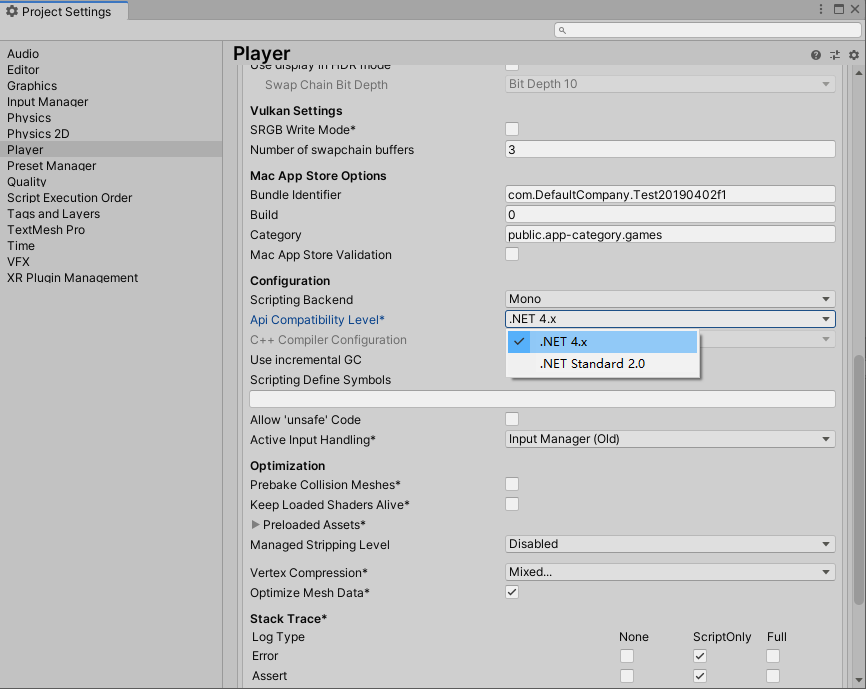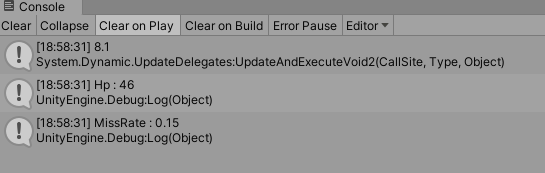# 汐夜资料

1.常用的值类型有：（struct）

2.常用的引用类型有：

string，class，array，delegate，interface

` 1 [System.Serializable]  2 public struct Property<T> where T : struct  3 {  4     public string Label { get; }  5     public T Value { get; }  6     public PropertyType Type { get; }  7     public Property(string label, T value, PropertyType type = PropertyType.Sub)  8     {  9         Label = label; 10         Value = value; 11         Type = type; 12     } 13  14     public static Property<T> operator +(Property<T> a, Property<T> b) 15     { 16         var prop = new Property<T>(); 17         if (a.Label == b.Label && a.Type == b.Type) 18         { 19             //怎么知道这个值到底是int还是float... 20         } 21         return prop; 22     } 23 }`

`1 public enum PropertyType 2 { 3     Main, 4     Sub 5 }`

` 1     public static Property<T> operator +(Property<T> a, Property<T> b)  2     {  3         if (a.Label == b.Label && a.Type == b.Type)  4         {  5             object tempa = a.Value;  6             object tempb = b.Value;  7   8             object add;  9             if (tempa is int) 10             { 11                 add = (int)tempa + (int)tempb; 12             } 13             else if (tempa is float) 14             { 15                 add = (float)tempa + (float)tempb; 16             } 17             //...其他类型 18             else 19             { 20                 return new Property<T>(); 21             } 22  23             return new Property<T>(a.Label, (T)add, a.Type); 24         } 25         return new Property<T>(); 26     }`

`1             if (tempa.GetType() == typeof(float)) 2             { 3  4             } 5             //or 6             if (typeof(T) == typeof(float)) 7             { 8  9             }`

.Net 4.0 以后开始支持动态数据类型——也就是dynamic关键字；令人兴奋的是，dynamic可以被赋值为任何一种类型的值，当然也包括泛型。

` 1     public static Property<T> operator +(Property<T> a, Property<T> b)  2     {  3         if (a.Label == b.Label && a.Type == b.Type)  4         {  5             dynamic x1 = a.Value;  6             dynamic x2 = b.Value;  7             return new Property<T>(a.Label, (T)(x1 + x2), a.Type);  8         }  9         return new Property<T>(); 10     }`

` 1     public static Property<T> operator +(Property<T> a, Property<T> b)  2     {  3         if (a.Label == b.Label && a.Type == b.Type)  4         {  5             if (typeof(T) != typeof(bool) && typeof(T)!=typeof(Enum))  6             {  7                 dynamic x1 = a.Value;  8                 dynamic x2 = b.Value;  9                 return new Property<T>(a.Label, (T)(x1 + x2), a.Type); 10             } 11         } 12         return new Property<T>(); 13     }`` 1 using UnityEngine;  2   3 public class MicrosoftCSharpTest : MonoBehaviour  4 {  5     void Start()  6     {  7         dynamic a = 5.1f;  8         dynamic b = 3;  9         Debug.Log(a + b); 10  11         var hp1 = new Property<int>("Hp", 41); 12         var hp2 = new Property<int>("Hp", 5); 13         var hp = hp1 + hp2; 14         Debug.Log(hp.Label + " : " + hp.Value); 15  16         var miss1 = new Property<float>("MissRate", .1f); 17         var miss2 = new Property<float>("MissRate", .05f); 18         var miss = miss1 + miss2; 19         Debug.Log(miss.Label + " : " + miss.Value); 20     } 21 }`qklbishe.com区块链毕设代做网专注|以太坊fabric-计算机|java|毕业设计|代做平台 » 汐夜资料